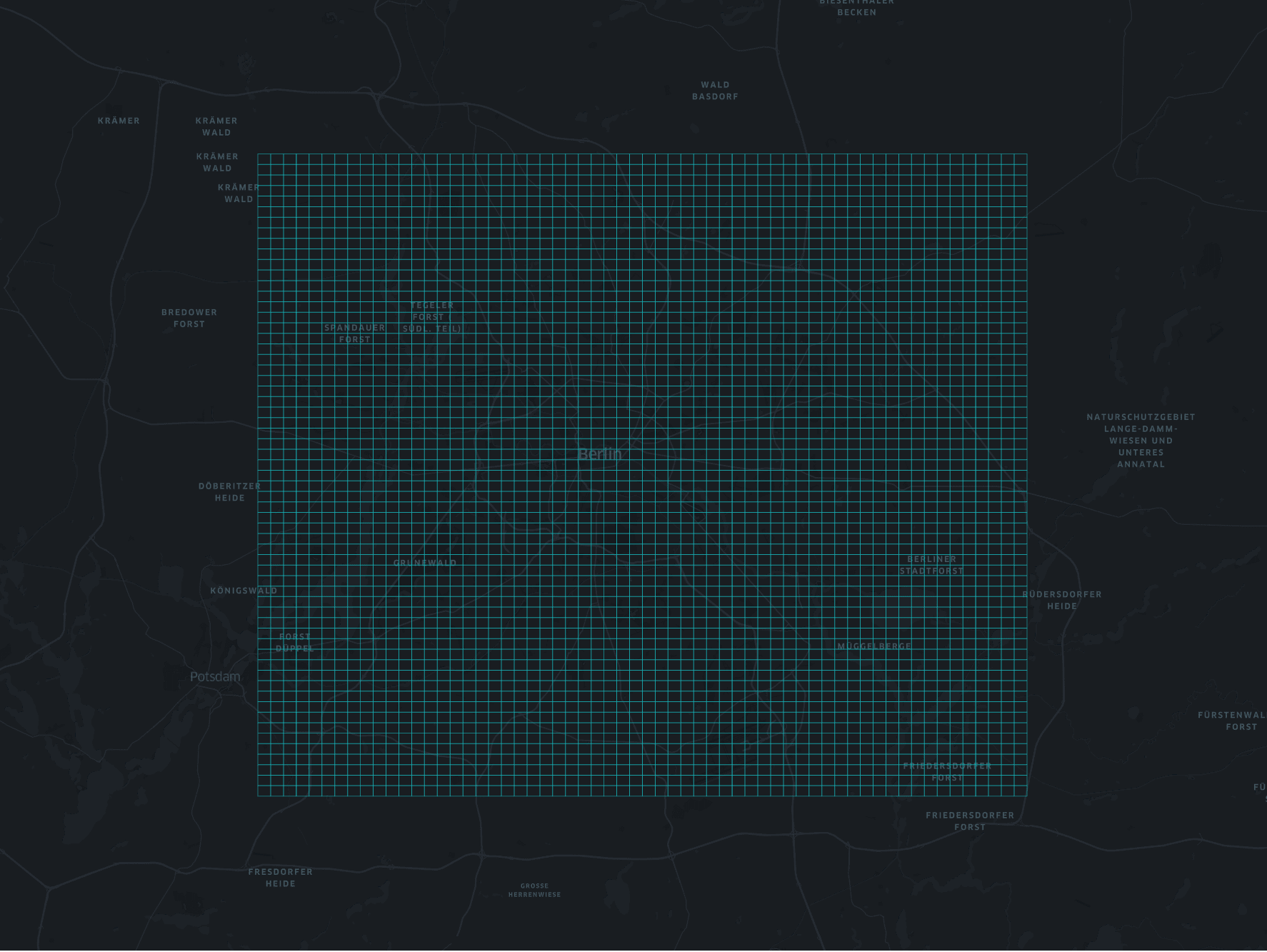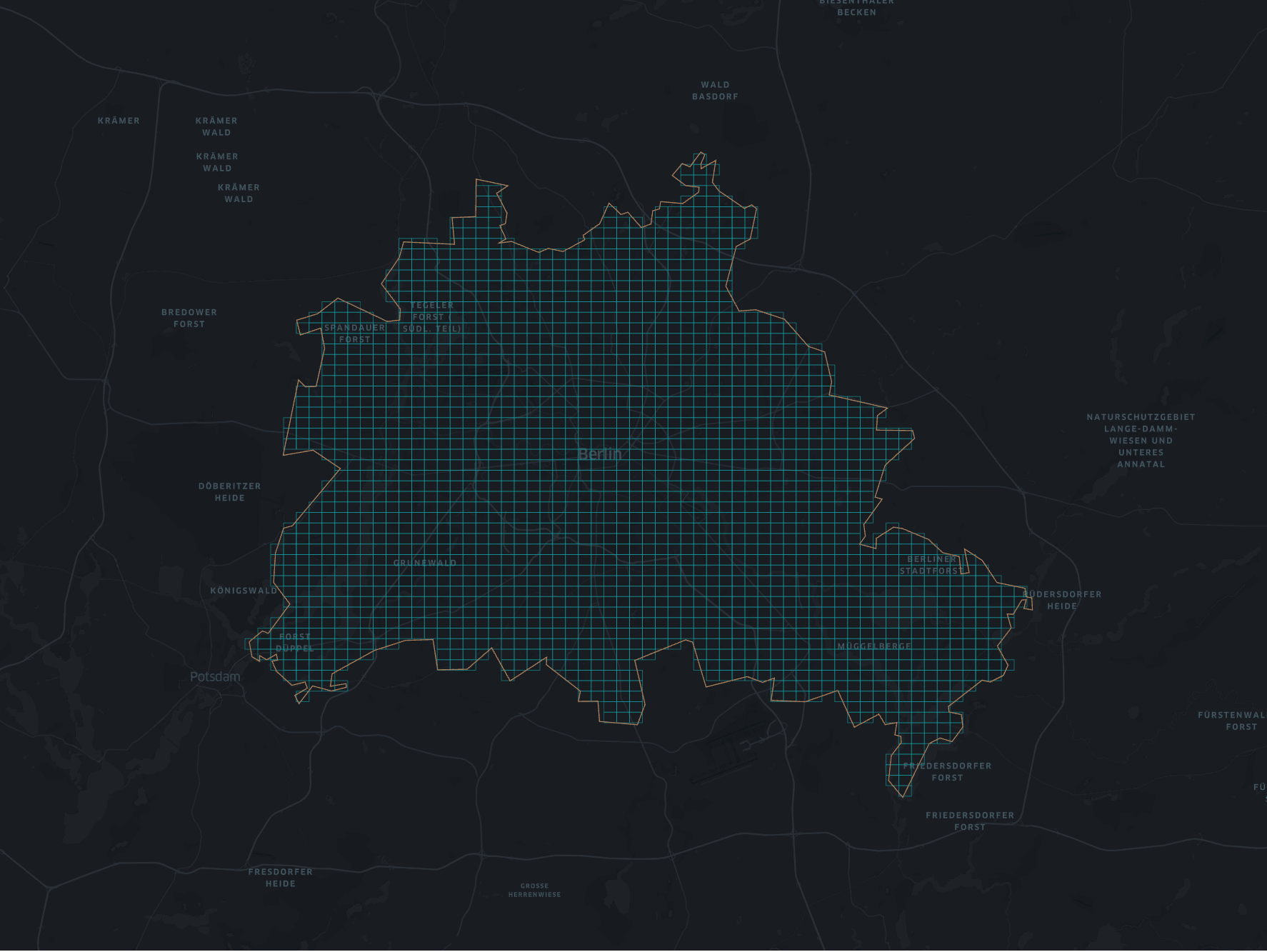When dealing with geospatial data it is sometimes useful to have a grid at hand that represents the given data. One way to create a grid like this is to use Geohashes. GeoHashes are a hierarchical spatial data structure which subdivides space into buckets of grid shape, which is one of the many applications of what is known as a Z-order curve, and generally space-filling curves. A Geohash is an encoded character string that is computed from geographic coordinates. The longer the Geohash the smaller is the rectangle it represents.

The following table shows the relationship between Geohash length and the size of the rectangle represented by that Geohash at the equator.

Length Tile Size
1 5,009.4km x 4,992.6km
2 1,252.3km x 624.1km
3 156.5km x 156km
4 39.1km x 19.5km
5 4.9km x 4.9km
12 3.7cm x 1.9cm

Let's say we want to populate a grid over Berlin at level 5 (4.9km x 4.9km). The bounding box for Berlin is SW (lat, lon) = 52.338261, 13.08835 and NE (lat, lon) = 52.67551, 13.76116. The algorithm to populate the given bounding box works as follows:

• Get roughly the center of the bounding box, compute the Geohash, save it and put it also on a stack
• While the stack is not empty
• pop one Geohash
• compute the 8 neighbors
• if they are inside the bounding box, save them and put them on the stack
• keep a record of already checked Geohashes to avoid unnecessary computation

The whole thing in Python:

``````import geohash

def is_geohash_in_bounding_box(current_geohash, bbox_coordinates):
"""Checks if the box of a geohash is inside the bounding box

:param current_geohash: a geohash
:param bbox_coordinates: bounding box coordinates
:return: true if the the center of the geohash is in the bounding box
"""

coordinates = geohash.decode(current_geohash)
geohash_in_bounding_box = (bbox_coordinates < coordinates < bbox_coordinates) and (
bbox_coordinates < coordinates < bbox_coordinates)
return geohash_in_bounding_box

def build_geohash_box(current_geohash):
"""Returns a GeoJSON Polygon for a given geohash

:param current_geohash: a geohash
:return: a list representation of th polygon
"""

b = geohash.bbox(current_geohash)
polygon = [(b['w'], b['s']), (b['w'], b['n']), (b['e'], b['n']), (b['e'], b['s'],), (b['w'], b['s'])]
return polygon

def compute_geohash_tiles(bbox_coordinates):
"""Computes all geohash tile in the given bounding box

:param bbox_coordinates: the bounding box coordinates of the geohashes
:return: a list of geohashes
"""

checked_geohashes = set()
geohash_stack = set()
geohashes = []
# get center of bounding box, assuming the earth is flat ;)
center_latitude = (bbox_coordinates + bbox_coordinates) / 2
center_longitude = (bbox_coordinates + bbox_coordinates) / 2

center_geohash = geohash.encode(center_latitude, center_longitude, precision=GEOHASH_PRECISION)
geohashes.append(center_geohash)
while len(geohash_stack) > 0:
current_geohash = geohash_stack.pop()
neighbors = geohash.neighbors(current_geohash)
for neighbor in neighbors:
if neighbor not in checked_geohashes and is_geohash_in_bounding_box(neighbor, bbox_coordinates):
geohashes.append(neighbor)
return geohashes
``````

In order to visualize the resulting grid it helps to convert the results to GeoJSON

``````import geojson
from geojson import MultiLineString

def write_geohash_layer(geohashes):
"""Writes a grid layer based on the geohashes

:param geohashes: a list of geohashes
"""

layer = MultiLineString([build_geohash_box(gh) for gh in geohashes])
with open('ghash_berlin_bbox.json', 'wb') as f:
f.write(geojson.dumps(ghash_polygon, sort_keys=True).encode('utf-8'))
``````

The result can be visualized with Kepler.gl.Sometimes a bounding box is not enought and you want a more specific shape for the grid. In this case you can use a GeoJSON polygon as an input. I downloaded a polygon of Berlin from OpenDataLab.

The algorithm is almost the same as above. The only difference is that we test if a point is inside the given polygon. Therefor, we use the `shapely` library

``````from shapely import geometry

def compute_geohash_tiles_from_polygon(polygon):
"""Computes all hex tile in the given polygon

:param polygon: the polygon
:return: a list of geohashes
"""

checked_geohashes = set()
geohash_stack = set()
geohashes = []
# get center of bounding, assuming the earth is flat ;)
center_latitude = polygon.centroid.coords
center_longitude = polygon.centroid.coords

center_geohash = geohash.encode(center_latitude, center_longitude, precision=GEOHASH_PRECISION)
geohashes.append(center_geohash)
while len(geohash_stack) > 0:
current_geohash = geohash_stack.pop()
neighbors = geohash.neighbors(current_geohash)
for neighbor in neighbors:
point = geometry.Point(geohash.decode(neighbor)[::-1])
if neighbor not in checked_geohashes and polygon.contains(point):
geohashes.append(neighbor)